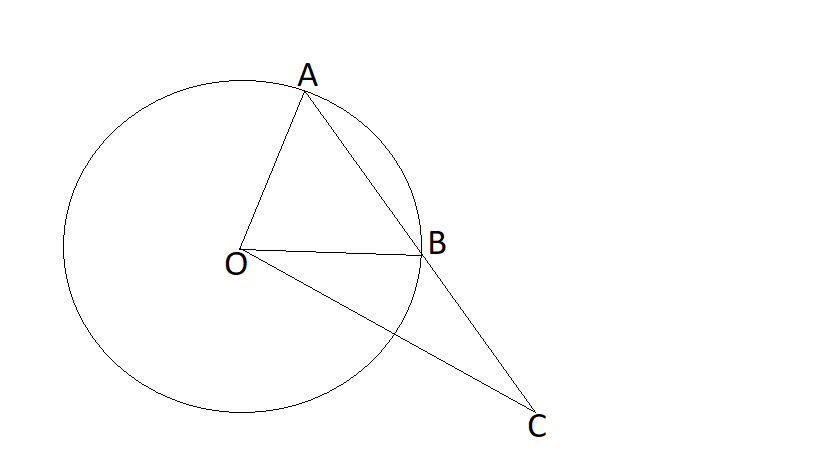# Got stuck at a point -1

While I was spending time on geometry and triangle congruence triangles a question came in my mind which I wasn't able to solve, it can be described as follows:

In the given figure $O$ is the center of the circle.If you only knows measure of $\overline{OA},\overline{OB}, \overline{OC}$ and $\angle ACO$ then can you find measure of $\angle AOB$ ?

Any answer will be worthy and will be appreciate, so please try once!Note by Zakir Husain
1 year, 1 month ago

This discussion board is a place to discuss our Daily Challenges and the math and science related to those challenges. Explanations are more than just a solution — they should explain the steps and thinking strategies that you used to obtain the solution. Comments should further the discussion of math and science.

When posting on Brilliant:

• Use the emojis to react to an explanation, whether you're congratulating a job well done , or just really confused .
• Ask specific questions about the challenge or the steps in somebody's explanation. Well-posed questions can add a lot to the discussion, but posting "I don't understand!" doesn't help anyone.
• Try to contribute something new to the discussion, whether it is an extension, generalization or other idea related to the challenge.

MarkdownAppears as
*italics* or _italics_ italics
**bold** or __bold__ bold
- bulleted- list
• bulleted
• list
1. numbered2. list
1. numbered
2. list
Note: you must add a full line of space before and after lists for them to show up correctly
paragraph 1paragraph 2

paragraph 1

paragraph 2

[example link](https://brilliant.org)example link
> This is a quote
This is a quote
    # I indented these lines
# 4 spaces, and now they show
# up as a code block.

print "hello world"
# I indented these lines
# 4 spaces, and now they show
# up as a code block.

print "hello world"
MathAppears as
Remember to wrap math in $$ ... $$ or $ ... $ to ensure proper formatting.
2 \times 3 $2 \times 3$
2^{34} $2^{34}$
a_{i-1} $a_{i-1}$
\frac{2}{3} $\frac{2}{3}$
\sqrt{2} $\sqrt{2}$
\sum_{i=1}^3 $\sum_{i=1}^3$
\sin \theta $\sin \theta$
\boxed{123} $\boxed{123}$

Sort by:

I will try, although I don't know much of geometry(circles and all that).

However, I think $OA$ and $OB$ are the same (radii), so only one needs to be specified.

- 1 year, 1 month ago

Yeah, That's right

- 1 year, 1 month ago

I tried it, but could not do it with the geometry I am taught till now. I think it really requires higher class geometry like the solutions of Aryan Sanghi and Jeff Giif.

- 1 year, 1 month ago

You can do it using Sine rule

$\frac{OB}{sin C}$ = $\frac{OC}{sin OBC}$

As we know, OB, Angle C and OC, we can find angle OBC

With this, we can find angle ABO with linear pair

Now, angle A is equal to Angle ABO as OA = OB

So, we can apply sum of anglea of triangles is 180° in triangle AOB to get angle AOB

- 1 year, 1 month ago

- 1 year, 1 month ago

Yes, I suppose you can! Given $\frac{sinA}{a}$=$\frac{sinB}{b}$, you can substitute values of angle ACO and side OA, so
$\frac{sin(angleACO)}{sideOA}=\frac{sin(angleOAC)}{sideOC}.$
Since OA & OB are the radii of a same circle, triangleOAB is an isosceles triangle, so angle AOB =
$180^\circ - 2\times \angle OAC.$

- 1 year, 1 month ago

- 1 year, 1 month ago

$\angle OAB^{\circ} = \angle OBA^{\circ}$

In $\triangle AOC$: $\dfrac{AO}{\sin{(ACO^{\circ})}} = \dfrac{OC}{\sin{(\angle OAC^{\circ})}} \implies OAC^{\circ} = \sin^{-1} {\bigg(\dfrac{OC}{AO \times {\sin{(ACO^{\circ})}}}\bigg) \cdot }$

\begin{aligned} \angle BOA^{\circ} &= 180^{\circ} - 2 (\angle OAC^{\circ}) \end{aligned}

- 1 year, 1 month ago

@Zakir Husain, this answer is just a modification from @Jeff Giff's problem. I don't know which of these inspired the other, but both are nice!

- 1 year, 1 month ago